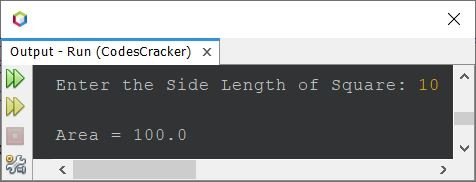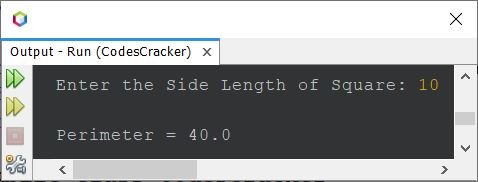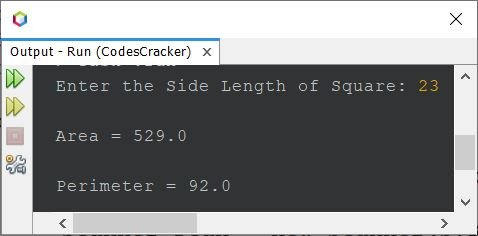# Java Program to Calculate Area and Perimeter of a Square

This article is created to cover a program in Java that calculates area and perimeter of a square based on the length of its side entered by user at run-time of the program.

Note - The area of a square is calculated using the formula s*s or s2. Where s is the length of side.

Note - The perimeter of a square is calculated using the formula 4*s.

## Find Area of Square in Java

The question is, write a program in Java to find and print area of a square. Following program is its answer:

```import java.util.Scanner;

public class CodesCracker
{
public static void main(String[] args)
{
float side, area;
Scanner s = new Scanner(System.in);

System.out.print("Enter the Side Length of Square: ");
side = s.nextFloat();

area = 4*side;
System.out.println("\nArea = " +area);
}
}```

The snapshot given below shows the sample run of above Java program with user input 10 as side length of a square whose area we want to see using the program:## Find Perimeter of Square in Java

The question is, write a program in Java to find and print perimeter of a square. The program given below is its answer:

```import java.util.Scanner;

public class CodesCracker
{
public static void main(String[] args)
{
float s, perimeter;
Scanner scan = new Scanner(System.in);

System.out.print("Enter the Side Length of Square: ");
s = scan.nextFloat();

perimeter = 4*s;
System.out.println("\nPerimeter = " +perimeter);
}
}```

The sample run of above program with same user input as of previous program, i.e., 10, is:## Calculate Area and Perimeter of Square in Java - Single Program

After combining both the program given above, this program is created, that calculates and prints the area and perimeter value both, of a square, whose side length is entered by user at run-time.

```import java.util.Scanner;

public class CodesCracker
{
public static void main(String[] args)
{
Scanner scan = new Scanner(System.in);

System.out.print("Enter the Side Length of Square: ");
float s = scan.nextFloat();

float a = s*s;
float p = 4*s;
System.out.println("\nArea = " +a);
System.out.println("\nPerimeter = " +p);
}
}```

The sample run of above program with user input 23 as side length of square is shown in the following snapshot:Note - For Area and Perimeter of Rectangle Program in Java, refer to its separate article.

#### Same Program in Other Languages

Java Online Test

« Previous Program Next Program »